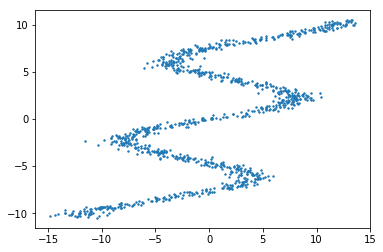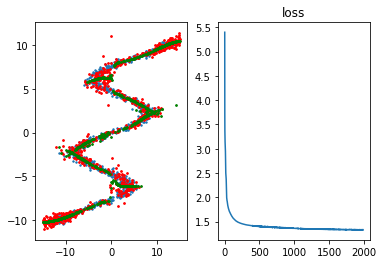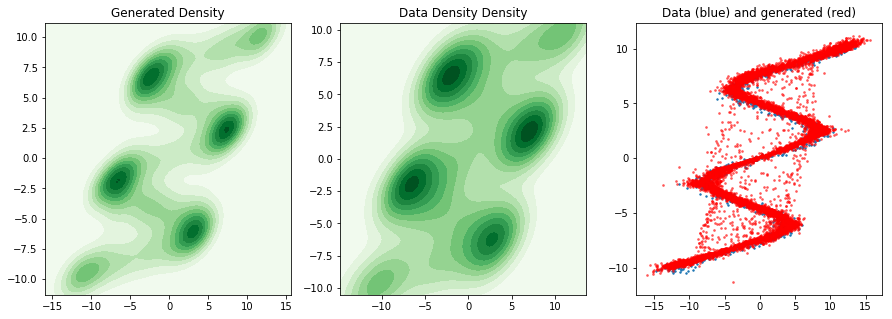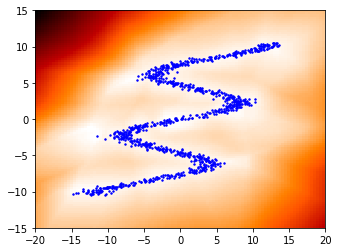# Gaussian mixture density networks in one dimension¶

Thomas Viehmann [email protected]

Today we want to implement a simple Gaussian mixture density network. Mixture Density Networks have been introduced by Bishop in the 1994 article of the same name.

The basic idea is simple: Given some input, say, $x$ we estimate the distribution of $Y | X=x$ as a mixture (in our case of Gaussians). In mixture density networks, the parameters of the mixture and its components are computed as a neural net. The canonical loss function is the negative log likelihood and we can backpropagate the loss through the parameters of the distribution to the network coefficients (so at the top there is what Kingma and Welling call the reparametrization trick and then there is plain neural network training).

Mixture density models are generative in the sense that you can sample from the estimated distribution $Y | X=x$.

Today we do this in 1d, but one popular application (in fact the one I implemented first) is the handwriting generation mode of A. Graves: Generating Sequences With Recurrent Neural Networks.

First, we import the world

In :
from matplotlib import pyplot
%matplotlib inline
import torch
import torch.utils.data
import numpy
from torch.autograd import Variable
import IPython
import itertools
import seaborn


I used David Ha's example which is almost twice as wiggly as Bishop's original example. Let's have a dataset and a data loader.

I will differ from Ha and Bishop in that I don't start with the case of the distribution to learn being the graph of a function of the condition $x$ (plus error), but we jump right into the non-graphical case.

In :
n_train = 1000
batch_size = 32
class DS(torch.utils.data.Dataset):
def __init__(self, n):
self.n = n
self.y = torch.rand(n)*21-10.5
self.x = torch.sin(0.75*self.y)*7.0+self.y*0.5+torch.randn(n)
def __len__(self):
return self.n
def __getitem__(self,i):
return (self.x[i],self.y[i])

train_ds = DS(n_train)
pyplot.scatter(train_ds.x.numpy(),train_ds.y.numpy(), s=2)
pyplot.show()
train_dl = torch.utils.data.DataLoader(train_ds, batch_size=batch_size)In :
class GaussianMixture1d(torch.nn.Module):
def __init__(self, n_in, n_mixtures, eps=0):
super(GaussianMixture1d, self).__init__()
self.n_in = n_in
self.eps = eps
self.n_mixtures = n_mixtures
self.lin = torch.nn.Linear(n_in, 3*n_mixtures)
self.log_2pi = numpy.log(2*numpy.pi)

def params(self, inp, pi_bias=0, std_bias=0):
# inp = batch x input
p = self.lin(inp)
pi = torch.nn.functional.softmax(p[:,:self.n_mixtures]*(1+pi_bias)) # mixture weights (probability weights)
mu = p[:,self.n_mixtures:2*self.n_mixtures] # means of the 1d gaussians
sigma = (p[:,2*self.n_mixtures:]-std_bias).exp() # stdevs of the 1d gaussians
sigma = sigma+self.eps
return pi,mu,sigma

def forward(self, inp, x):
# x = batch x 3 (=movement x,movement y,end of stroke)
# loss, negative log likelihood
pi,mu,sigma = self.params(inp)
log_normal_likelihoods =  -0.5*((x.unsqueeze(1)-mu) / sigma)**2-0.5*self.log_2pi-torch.log(sigma) # batch x n_mixtures
log_weighted_normal_likelihoods = log_normal_likelihoods+pi.log() # batch x n_mixtures
maxes,_ = log_weighted_normal_likelihoods.max(1)
mixture_log_likelihood = (log_weighted_normal_likelihoods-maxes.unsqueeze(1)).exp().sum(1).log()+maxes # log-sum-exp with stabilisation
neg_log_lik = -mixture_log_likelihood
return neg_log_lik

def predict(self, inp, pi_bias=0, std_bias=0):
# inp = batch x n_in
pi,mu,sigma = self.params(inp, pi_bias=pi_bias, std_bias=std_bias)
x = inp.data.new(inp.size(0)).normal_()
mixture = pi.data.multinomial(1)       # batch x 1 , index to the mixture component
sel_mu = mu.data.gather(1, mixture).squeeze(1)
sel_sigma = sigma.data.gather(1, mixture).squeeze(1)
x = x*sel_sigma+sel_mu
return Variable(x)

class Model(torch.nn.Module):
def __init__(self, n_inp = 1, n_hid = 24, n_mixtures = 24):
super(Model, self).__init__()
self.lin = torch.nn.Linear(n_inp, n_hid)
self.mix = GaussianMixture1d(n_hid, n_mixtures)
def forward(self, inp, x):
h = torch.tanh(self.lin(inp))
l = self.mix(h, x)
return l.mean()
def predict(self, inp, pi_bias=0, std_bias=0):
h = torch.tanh(self.lin(inp))
return self.mix.predict(h, std_bias=std_bias, pi_bias=pi_bias)

In :
m = Model(1, 32, 20)
opt = torch.optim.Adam(m.parameters(), 0.001)
m.cuda()
losses = []
for epoch in range(2000):
thisloss  = 0
for i,(x,y) in enumerate(train_dl):
x = Variable(x.float().unsqueeze(1).cuda())
y = Variable(y.float().cuda())
opt.zero_grad()
loss = m(x, y)
loss.backward()
thisloss += loss.data/len(train_dl)
opt.step()
losses.append(thisloss)
if epoch % 10 == 0:
IPython.display.clear_output(wait=True)
print (epoch, loss.data)
x = Variable(torch.rand(1000,1).cuda()*30-15)
y = m.predict(x)
y2 = m.predict(x, std_bias=10)
pyplot.subplot(1,2,1)
pyplot.scatter(train_ds.x.numpy(),train_ds.y.numpy(), s=2)
pyplot.scatter(x.data.cpu().squeeze(1).numpy(), y.data.cpu().numpy(),facecolor='r', s=3)
pyplot.scatter(x.data.cpu().squeeze(1).numpy(), y2.data.cpu().numpy(),facecolor='g', s=3)
pyplot.subplot(1,2,2)
pyplot.title("loss")
pyplot.plot(losses)
pyplot.show()

1990 1.3907438516616821Not bad! The green line basically gives the means of the mixture components rather than drawing from the mixture. This is implemented by biasing the variance. In some applications we might want to "clean" the output but not disable stochasticity completely. This can be achieved by biasing the variance of the components and biasing the component probabilites towards the largest one in the softmax. I learnt this trick from Gaves' article.

## Alternatives? Trying a GAN approach.¶

We might also try to reproduce a SLOGAN (Single sided Lipschitz Objective General Adversarial Network, a Wasserstein GAN variant). Note that while the MDN above tries to learn the distribution of $y$ given $x$, the GAN below tries to learn the unconditional distribution of pairs $(x,y)$.

I wrote a bit about the Wasserstein GAN and SLOGAN in two blog posts.

In :
class G(torch.nn.Module):
def __init__(self, n_random=2, n_hidden=50):
super(G, self).__init__()
self.n_random = n_random
self.l1 = torch.nn.Linear(n_random,n_hidden)
self.l2 = torch.nn.Linear(n_hidden,n_hidden)
#self.l2b = torch.nn.Linear(n_hidden,n_hidden)
self.l3 = torch.nn.Linear(n_hidden,2)
def forward(self, batch_size=32):
x = Variable(self.l1.weight.data.new(batch_size, self.n_random).normal_())
x = torch.nn.functional.relu(self.l1(x))
x = torch.nn.functional.relu(self.l2(x))
#x = torch.nn.functional.relu(self.l2b(x))
x = self.l3(x)
return x

class D(torch.nn.Module):
def __init__(self, lam=10.0, n_hidden=50):
super(D, self).__init__()
self.l1 = torch.nn.Linear(2,n_hidden)
self.l2 = torch.nn.Linear(n_hidden,n_hidden)
self.l3 = torch.nn.Linear(n_hidden,1)
self.one = torch.FloatTensor().cuda()
self.mone = torch.FloatTensor([-1]).cuda()
self.lam = lam
def forward(self, x):
x = torch.nn.functional.relu(self.l1(x))
x = torch.nn.functional.relu(self.l2(x))
x = self.l3(x)
return x
def slogan_loss_and_backward(self, real, fake):
self.zero_grad()
f_real = self(real.detach())
f_real_sum = f_real.sum()
f_real_sum.backward(self.one, retain_graph=True)
f_fake = self(fake.detach())
f_fake_sum = f_fake.sum()
f_fake_sum.backward(self.mone, retain_graph=True)
f_mean = (f_fake_sum+f_real_sum)
f_mean.abs().backward(retain_graph=True)
dist = ((real.view(real.size(0),-1).unsqueeze(0)-fake.view(fake.size(0),-1).unsqueeze(1))**2).sum(2)**0.5
f_diff = (f_real.unsqueeze(0)-f_fake.unsqueeze(1)).squeeze(2).abs()
lip_dists = f_diff/(dist+1e-6)
lip_penalty = (self.lam * (lip_dists.clamp(min=1)-1)**2).sum()
lip_penalty.backward()
return f_real_sum.data,f_fake_sum.data,lip_penalty.data, lip_dists.data.mean()

d = D()
d.cuda()
g = G(n_random=256, n_hidden=128)
g.cuda()
opt_d = torch.optim.Adam(d.parameters(), lr=1e-3)
opt_g = torch.optim.Adam(g.parameters(), lr=1e-3)

for p in itertools.chain(d.parameters(), g.parameters()):
if p.data.dim()>1:
torch.nn.init.orthogonal(p, 2.0)

In :
def endless(dl):
while True:
for i in dl:
yield i

batch_size=256
train_dl = torch.utils.data.DataLoader(train_ds, batch_size=batch_size)
train_iter = endless(train_dl)

for i in range(30001):
if i>0 and i%10000 == 0:
for pg in opt_g.param_groups:
pg['lr'] /= 3
for pg in opt_d.param_groups:
pg['lr'] /= 3
print ("new lr",pg['lr'])
for j in range(100 if i < 10 or i % 100 == 0 else 10):
g.eval()
d.train()
for p in d.parameters():
p.requires_grad = True
real_x,real_y = next(train_iter)
real = Variable(torch.stack([real_x.float().cuda(), real_y.float().cuda()],dim=1))
fake = g(batch_size=batch_size)
l_r, l_f, l_lip, lip_mean = d.slogan_loss_and_backward(real, fake)
opt_d.step()
if i % 100 == 0:
print ("f_r:",l_r,"f_fake:",l_f, "lip loss:", l_lip, "lip_mean", lip_mean)
g.train()
d.eval()
for p in d.parameters():
p.requires_grad = False
g.zero_grad()
fake = g(batch_size=batch_size)
f = d(fake)
fsum = f.sum()
fsum.backward()
opt_g.step()
if i % 1000 == 0:
IPython.display.clear_output(wait=True)
print (i)
fake = g(batch_size=10000)
fd = fake.data.cpu().numpy()
pyplot.figure(figsize=(15,5))
pyplot.subplot(1,3,1)
pyplot.title("Generated Density")
seaborn.kdeplot(fd[:,0], fd[:,1], shade=True, cmap='Greens', bw=0.01)
pyplot.subplot(1,3,2)
pyplot.title("Data Density Density")
seaborn.kdeplot(train_ds.x.numpy(),train_ds.y.numpy(), shade=True, cmap='Greens', bw=0.01)
pyplot.subplot(1,3,3)
pyplot.title("Data (blue) and generated (red)")
pyplot.scatter(train_ds.x.numpy(),train_ds.y.numpy(), s=2)
pyplot.scatter(fd[:,0], fd[:,1], facecolor='r',s=3, alpha=0.5)
pyplot.show()

30000We can try to see where $f$ sees the most discriminative power between the real sample and the generated fake distribution. Note that as $f$ depends on both distributions, it is not as simple as $f$ is low on the real data.

While we are far from perfectly resolving the path, it does not look too bad, either.

In :
N_dim = 100
x_test = torch.linspace(-20,20, N_dim)
y_test = torch.linspace(-15,15, N_dim)
x_test = (x_test.unsqueeze(0)*torch.ones(N_dim,1)).view(-1)
y_test = (y_test.unsqueeze(1)*torch.ones(1,N_dim)).view(-1)
xy_test = Variable(torch.stack([x_test, y_test], dim=1).cuda())
f_test = d(xy_test)
pyplot.imshow(f_test.data.view(N_dim,N_dim).cpu().numpy(), origin='lower', cmap=pyplot.cm.gist_heat_r, extent=(-20,20,-15,15))
pyplot.scatter(train_ds.x.numpy(),train_ds.y.numpy(), facecolor='b', s=2);Update: In a previous version, I expressed discontent with the GAN result. I revisited this notebook and after playing with the learning rate and increasing the noise dimension, the GAN seems to work reasonably well, too.

I appreciate your feedback at [email protected].

In [ ]: